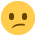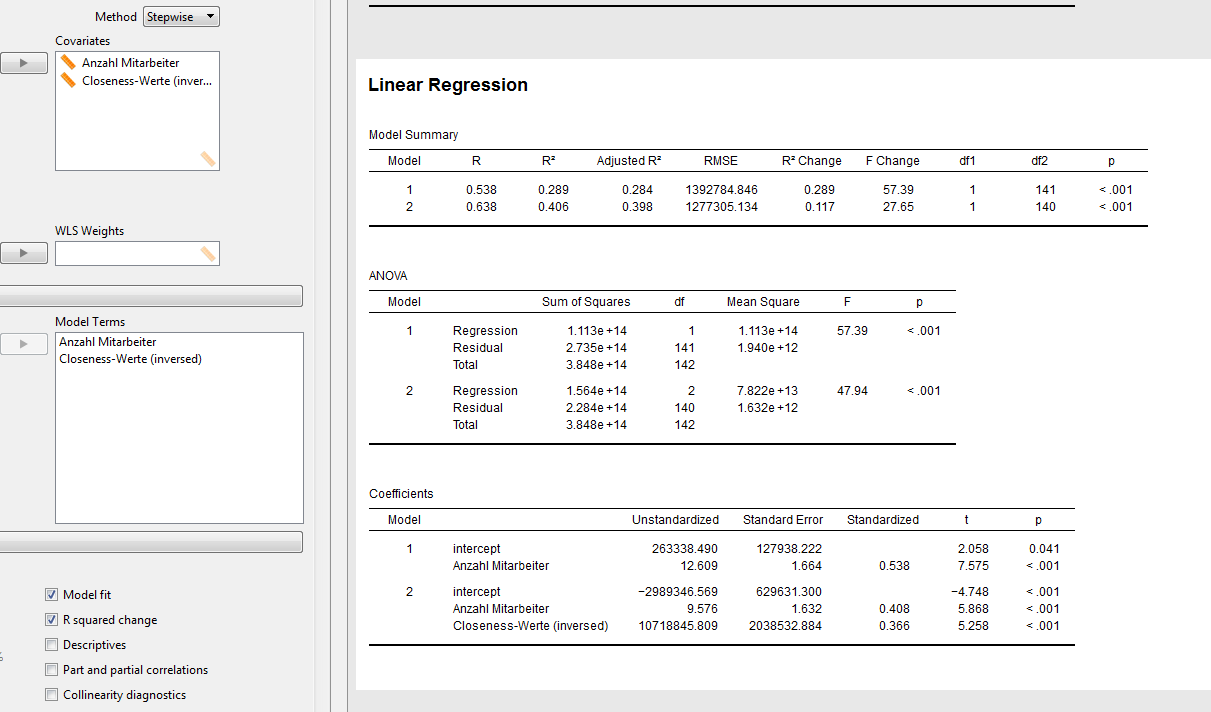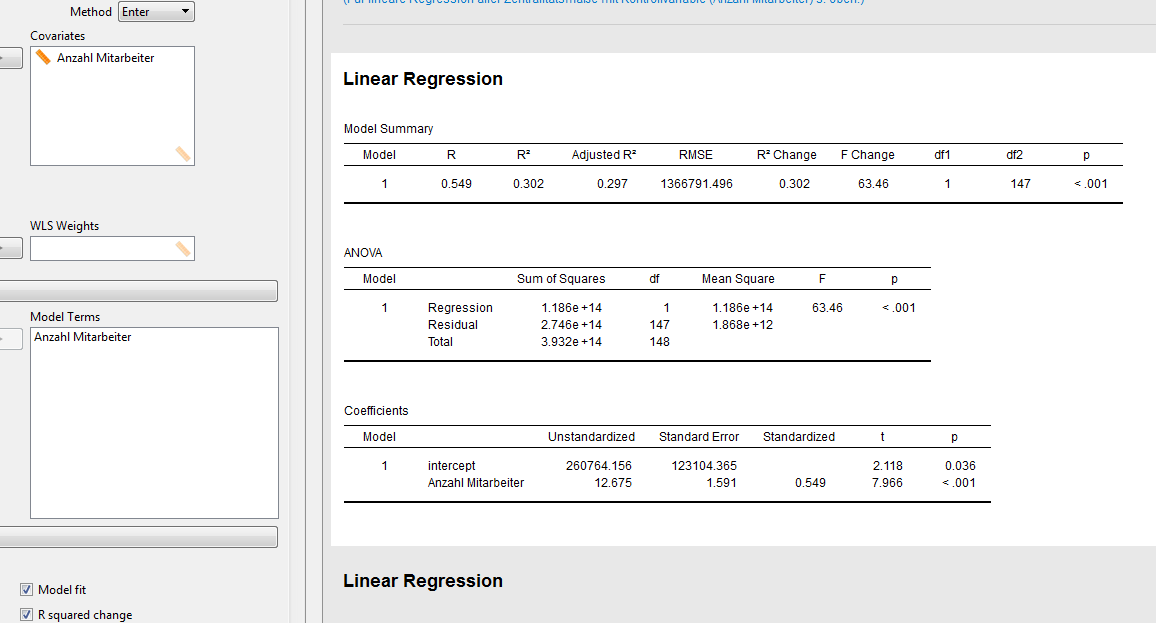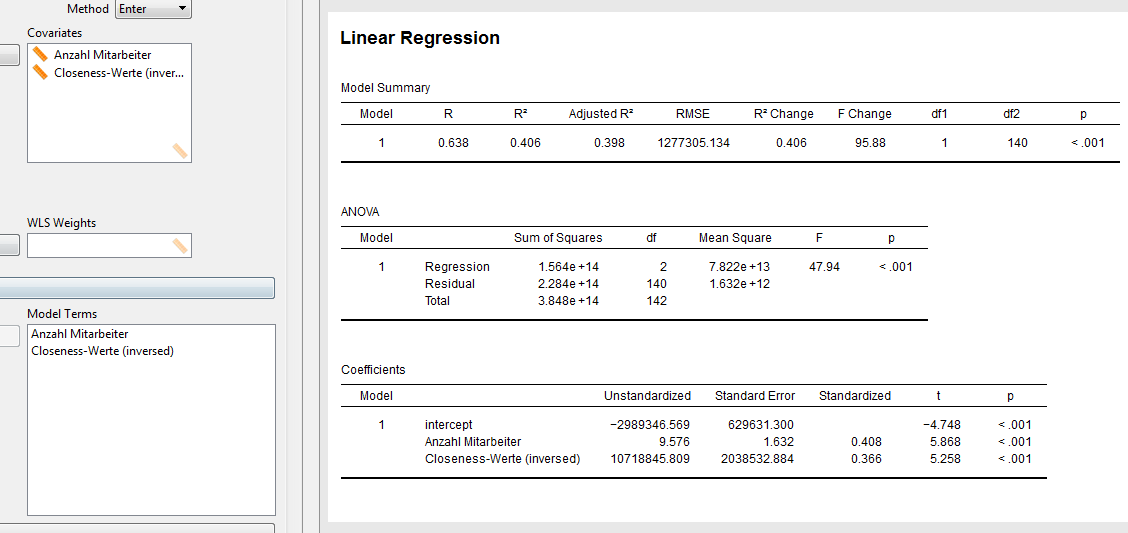#### Howdy, Stranger!

It looks like you're new here. If you want to get involved, click one of these buttons!

Supported by

# Stepwise hierarchical regression

edited September 2016

When I do a hierarchical regression, on one occasion I am not able to choose which variable is computed in the first block and which is added in the second block. It is automatically decided and I don't seem to be able to change that order by removing and re-adding the covariates. Any ideas on how I can solve this problem?

• hi Jannik,

Can you send along a .jasp file or some screenshots so we can reproduce this?

Cheers,
E.J.

• hi EJ,

I can't send you the actual file but a screenshot sure!

• edited September 2016

Basically I'd like to go "Anzahl Mitarbeiter" into the first block and then add "Degree-Werte" for the second block. What's strange is that when I did the same thing for a different independent variable to add into block 2, it was no problem.

e to clarify:
I first added "Anzahl Mitarbeiter" which worked fine. When I then added "Degree-Werte", "Degree-Werte" was automatically added into block 1 and the effect of "Anzahl Mitarbeiter" was added in block 2 (also see screenshot in the post above).

• OK, I'll forward this to our stepwise regression expert :-)

• Thx, I appreciate it!

• Hi Jannik,

Looking at your screenshot, it seems to me that you have specified "method = Stepwise" which is the
reason why variable inclusion/exclusion cannot be changed manually but is done automatically.
In case you want to have full control of the variables that enter the model, you could use "method = Enter".
However, at the moment, it is not possible to specify multiple blocks (i.e., what some people call "hierarchical regression") when using the Enter method. There is a feature request on our Github page: https://github.com/jasp-stats/jasp-desktop/issues/1418, so it is on our list.

Cheers,
Quentin

• edited September 2016

Hi Quentin,

Yes, apparently I was looking for the not yet existent featureI needed to do it this way to calculate the R² change when adding the additional effect. Is it possible to manually (with a calculator/excel) calculate R² change from the R² of a model 1 with only the control variable and the R² of a model with both the control and the needed independent variable?

How is the automatic inclusion/exclusion determined? Is it possible to influence the order by doing something in the orgininal data sheet?

• Hi Jannik,

Yes, you can manually calculate R-squared change: simply substract the R-squared value for the model with less predictor variables from the one for the model with more predictor variables (i.e., in your case, substract the R-squared value for model 1 from the one for the model with the control and needed independent variables).

When using the "Stepwise" method, an initial model is defined that only contains the constant. Then, from the set of possible predictors, successively the one is added that best predicts the dependent variable (you can select which criterion is used for this by going to "Options->Stepping Method Criteria"). After a predictor has been added, a removal test is made to see whether any redundant predictors can be removed (obviously, this is only interesting for the predictors that have been added in a previous step, not the one that has just been added). Again, you can select which criterion is used for this by going to "Options->Stepping Method Criteria". When no further predictor can be added or removed according to the specified criteria, the algorithm stops.

If your last question refers to whether you can change the data file in such a way that you obtain the test that you wish to conduct, I am afraid the answer is no. However, of course, if you change your data in that file, the algorithm might possibly produce a different result (but this is probably not interesting, since you are now analyzing a different data set).

Cheers,
Quentin

• edited September 2016

Hi Quentin,

Thx for the explanations.

To just calculate R² change by substracting does not seem to work as Jasp calculates different values of R² for
case a) performing a stepwise calculation and let Jasp calculate the R² change
case b) performing two separate calculations for model 1 (only control variable) and model 2 (control variable + independent variable)

case a) gives R² change = 0,406 - 0,289 = 0,117
case b) gives R² change = 0,406 - 0,302 = 0,104
This seems to be an issue.

Also see screenshots attached.• Hi Jannik,

Looking at the output, the first thing I notice is that the regression coefficient for "Anzahl Mitarbeiter" differs between the "Stepwise" model 1 (i.e., 12.609) and the "Enter" model 1 (i.e., 12.675). Could you mabye send me the data file to quentingronau@web.de so that I can try to figure out whether this is a bug in JASP?
Thanks!

• Hi Quentin,

I'm sorry but as it's not my data and I was explicitly told not to give it out, I can't.

If I may still ask another question. I realized that Jasp gives out p-values at the end of the line in the coefficients block of the results. This would be the p-value of the standardized coefficient right? Is it possible to display p-values for the unstandardized coefficients also?

• Hi Jannik,

I understand, no worries!
In case you can reproduce this behavior with another data set that you can share, we would appreciate if you could let us know.
With respect to your question: the p-values for the standardized and unstandardized coefficients are the same.

Cheers,
Quentin